## 6.2 Iterate something from a non attainable subdemonstration

Inside the main demonstration (which goes from the first line to the last), we can open child demonstrations (subdemonstrations). Inside any subdemonstration we can also have a subsubdemonstration, which would have as father the subdemonstration and as grandfather the main demonstration.

To understand this, here is the solved example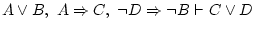: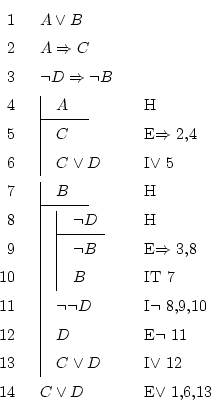Well, any demonstration can only access the formulas from inside itself, inside its father, inside the father of its father, inside the father of the father of its father, ... All these are called ancestors, so: a demonstration can access itself and its ancestors.

For this reason, it we are on line 10, the derivation rules can use formulas from the following places:

• the current demonstration (lines 8 and 9 currently).
• father demonstration of the 8-10 one, so, from line 7.
• from the demonstration father of the one which starts at line 7, that's it, lines 1 to 3.
Bet never we could use the formulas from lines 4 to 6, which is the demonstration uncle of the current one (brother of its father), because all that demonstration is based on the hypothesis that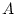(line 4), and we're not doing that supposition anymore.

In logical language, one says that a formulais actual at formula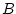if being inwe can use. For this to be true,must have been written before, and some ancestor ofmust be father of.

So, to prove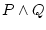we can't do this: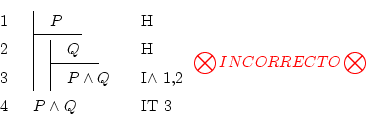Daniel Clemente Laboreo 2005-05-17